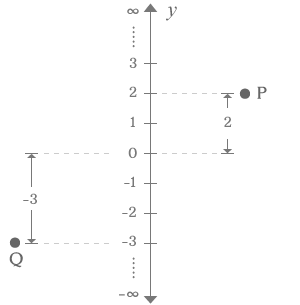y-coordinate

The distance of a point from the origin in the point of view of y-axis is called y-coordinate.

The vertical y-axis has a number line and it is used to determine the distance of a point. The distance of a point is actually determined by comparing its distance from origin with the distances on the number line of y-axis but the distance is measured in the direction parallel to the y-axis. The distance of the point in the point of view of y-axis is y-coordinate of the point.

ExampleP and Q are two points. The vertical distance of each point is y-coordinate to the respective point. Actually, the vertical distance of each point is the distance parallel to the y-axis.

The Point P is exactly at division 2 in the point of y-axis. The distance of the point P from origin of y-axis to division 2 is 2. Therefore, y-coordinate of the point P is 2.

The point Q is exactly at division -3 in the point of y-axis. The distance of the point Q from origin of y-axis to division -3 is -3. So, the y-coordinate of the point Q is -3.

The distances are measured in parallel direction of y-axis to determine the y-coordinate of points P and Q in Cartesian coordinate system.

Latest Math Topics
Latest Math Problems
Email subscription
Math Doubts is a best place to learn mathematics and from basics to advanced scientific level for students, teachers and researchers. Know more